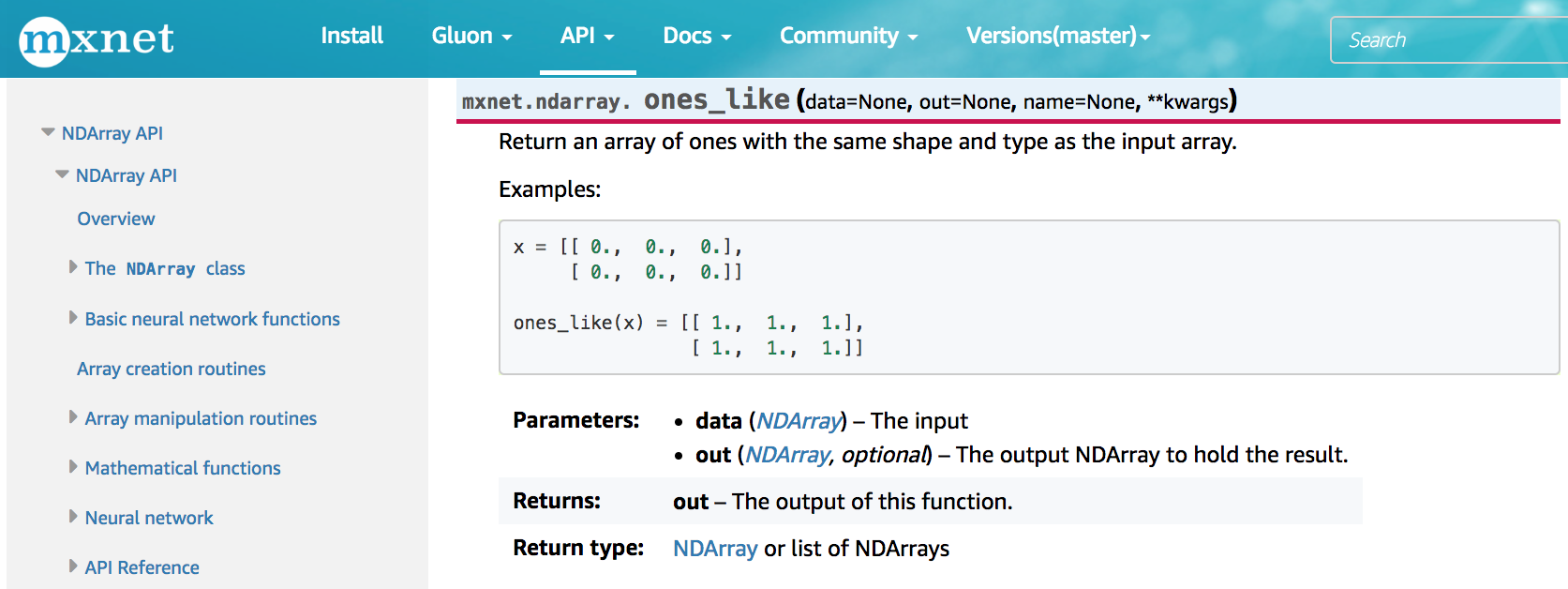# 2.4. 查阅文档¶

## 2.4.1. 查找模块里的所有函数和类¶

In :

from mxnet import nd

print(dir(nd.random))

['NDArray', '_Null', '__all__', '__builtins__', '__cached__', '__doc__', '__file__', '__loader__', '__name__', '__package__', '__spec__', '_internal', '_random_helper', 'current_context', 'exponential', 'exponential_like', 'gamma', 'gamma_like', 'generalized_negative_binomial', 'generalized_negative_binomial_like', 'multinomial', 'negative_binomial', 'negative_binomial_like', 'normal', 'normal_like', 'numeric_types', 'poisson', 'poisson_like', 'randint', 'randn', 'shuffle', 'uniform', 'uniform_like']


## 2.4.2. 查找特定函数和类的使用¶

In :

help(nd.ones_like)

Help on function ones_like:

ones_like(data=None, out=None, name=None, **kwargs)
Return an array of ones with the same shape and type
as the input array.

Examples::

x = [[ 0.,  0.,  0.],
[ 0.,  0.,  0.]]

ones_like(x) = [[ 1.,  1.,  1.],
[ 1.,  1.,  1.]]

Parameters
----------
data : NDArray
The input

out : NDArray, optional
The output NDArray to hold the result.

Returns
-------
out : NDArray or list of NDArrays
The output of this function.



In :

x = nd.array([[0, 0, 0], [2, 2, 2]])
y = x.ones_like()
y

Out:


[[1. 1. 1.]
[1. 1. 1.]]
<NDArray 2x3 @cpu(0)>


## 2.4.3. 在MXNet网站上查阅¶## 2.4.4. 小结¶

• 遇到不熟悉的MXNet API时，可以主动查阅它的相关文档。
• 查阅MXNet文档可以使用dirhelp函数，或访问MXNet官方网站。

## 2.4.5. 练习¶

• 查阅NDArray支持的其他操作。

## 2.4.6. 扫码直达讨论区¶